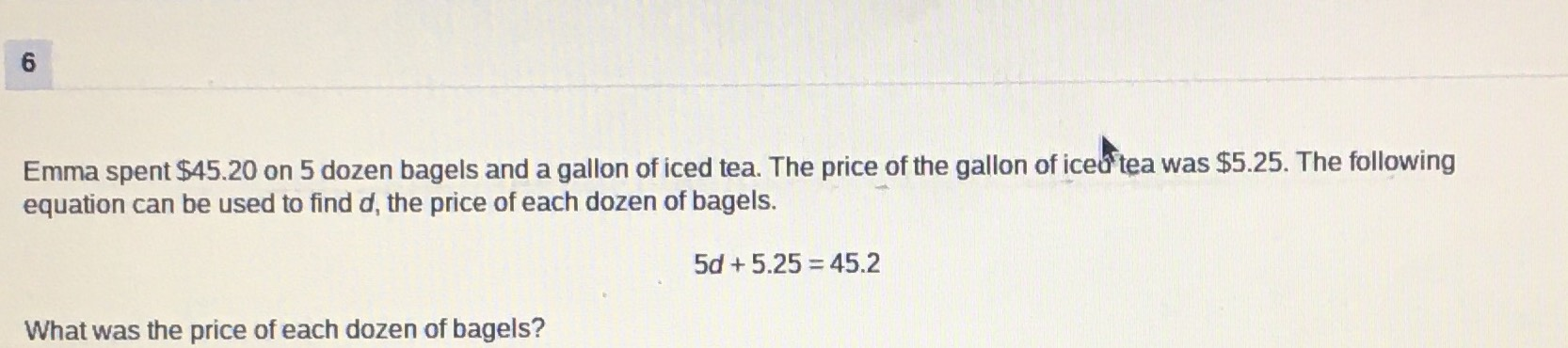### ¿Todavía tienes preguntas de matemáticas?

Pregunte a nuestros tutores expertos
Algebra
Pregunta$$6$$ Emma spent $$\ 45.20$$ on $$5$$ dozen bagels and a gallon of iced tea. The price of the gallon of iceut tea was $$\ 5.25$$ . The following equation can be used to find $$d$$ , the price of each dozen of bagels.

$$5 d + 5.25 = 45.2$$